If fractions make you want to scream, try this quiz to help you learn.

# Fractions 2

This Math quiz is called 'Fractions 2' and it has been written by teachers to help you if you are studying the subject at middle school. Playing educational quizzes is a fabulous way to learn if you are in the 6th, 7th or 8th grade - aged 11 to 14.

It costs only \$12.50 per month to play this quiz and over 3,500 others that help you with your school work. You can subscribe on the page at Join Us

Fractions are all about splitting up a whole item into smaller, equal size parts. If you have completed the quizzes on Decimals or Percentages then you will already have come across two types of fractions. This middle school Math quiz will test how well you deal with the third type, common fractions.

A common fraction has two parts to it – the denominator, or bottom number, tells you how many equal parts one whole unit is split into. The numerator, or top number, indicates how many parts you have. A horizontal fraction bar is used to separate the numerator from the denominator – don’t be tempted to use the / symbol, as this can lead to mistakes when working with fractions.

There are four techniques you should be able to apply to fractions.

The first is finding equivalent fractions – multiply (or divide) both the numerator and denominator by the same number. if you are asked to cancel down or simplify a fraction, ask yourself what number goes into both the top and the bottom, and divide by it.

Adding and subtracting fractions require you to find equivalent fractions, so that they both have the same denominator. Then you simply add (or subtract) the numerator.

Multiplying fractions is perhaps the easiest operation – change any mixed numbers to top heavy fractions, then multiply the top row, and multiply the bottom row. Always looks to see if you can cancel down.

Dividing fractions is similar to multiplying, except you invert the second fraction, and change the divide sign to multiply.

The root of the word FRACTION comes from the Latin ‘frac’, which means to break. Will you be broken by fractions?

1.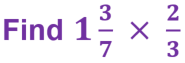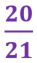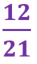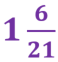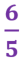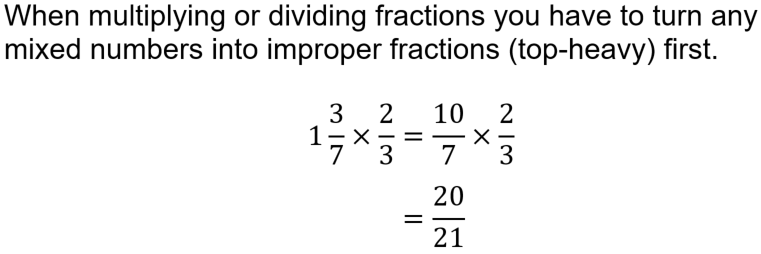2.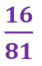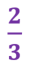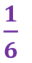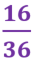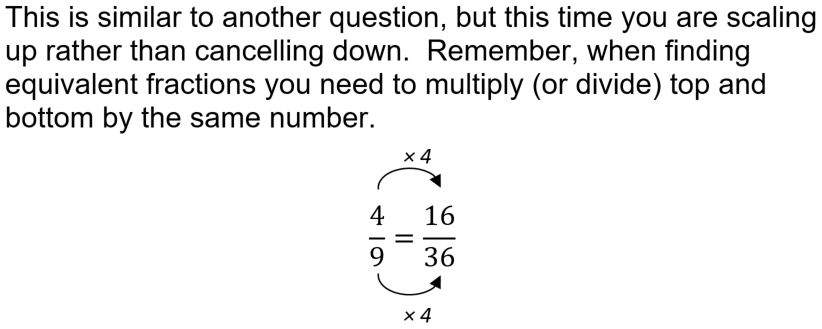3.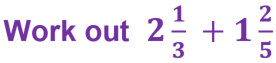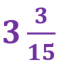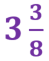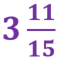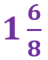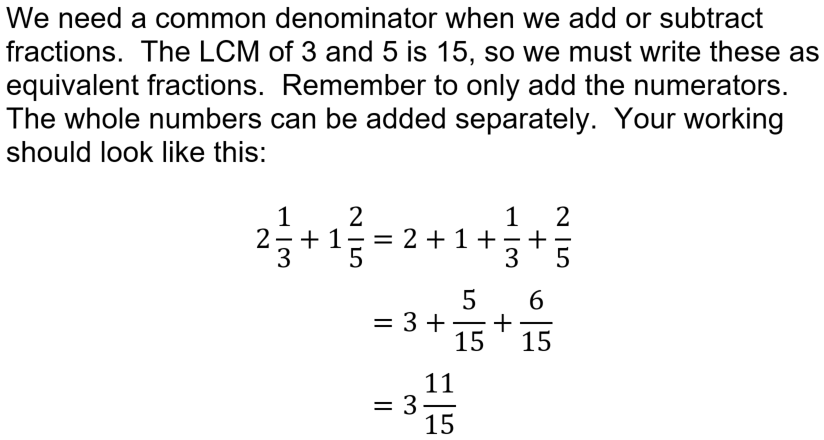4.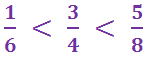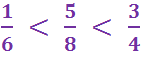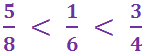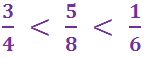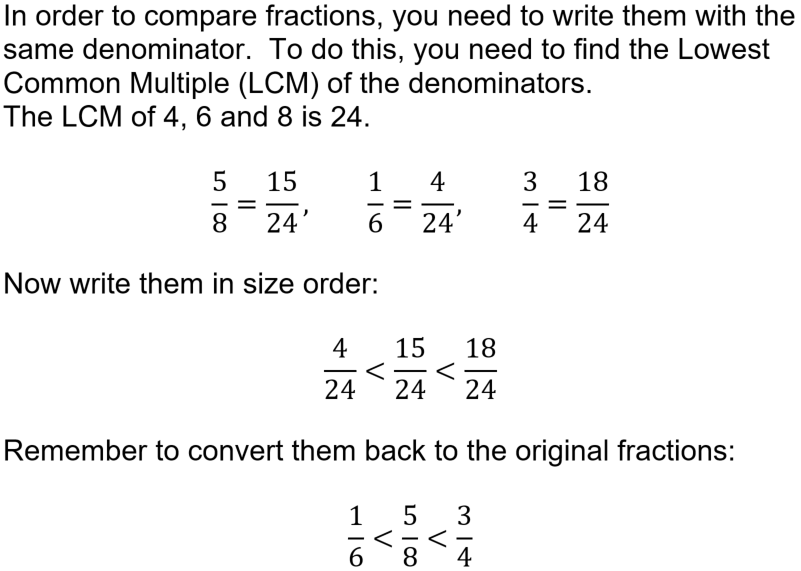5.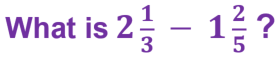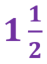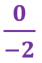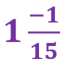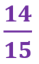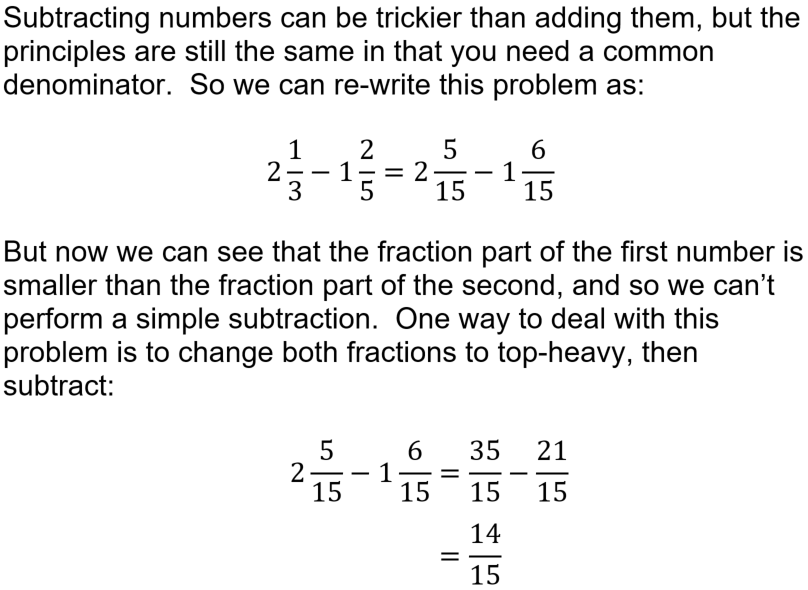6.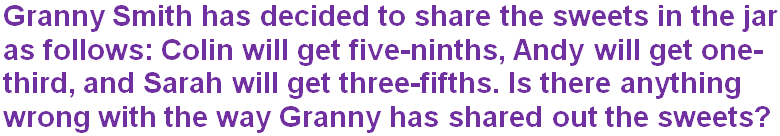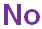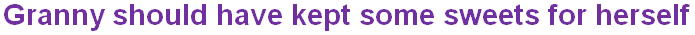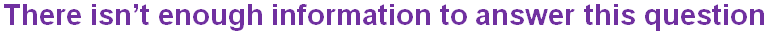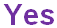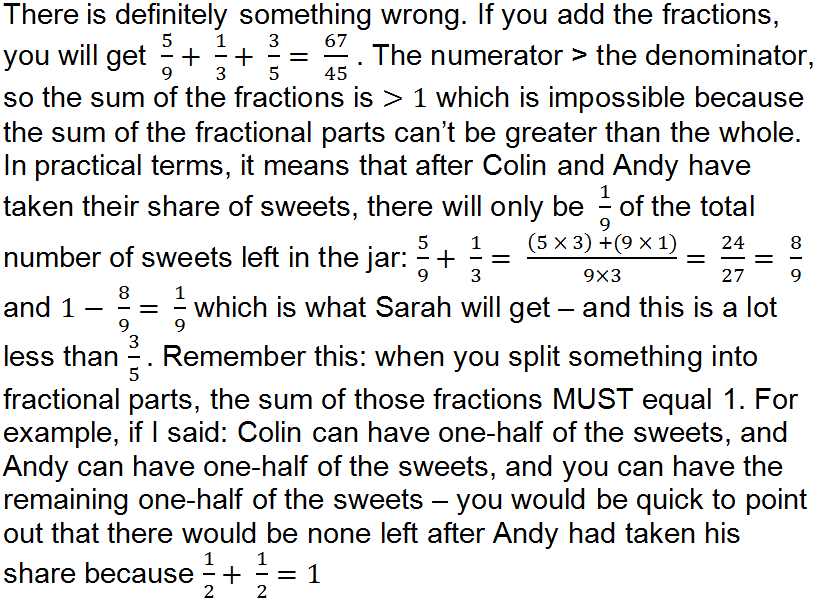7.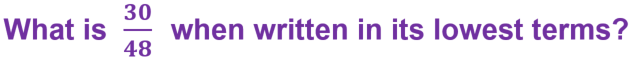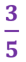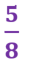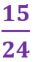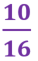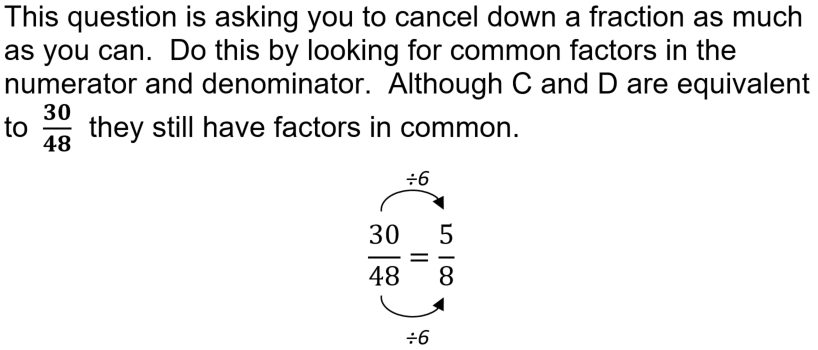8.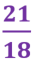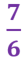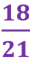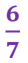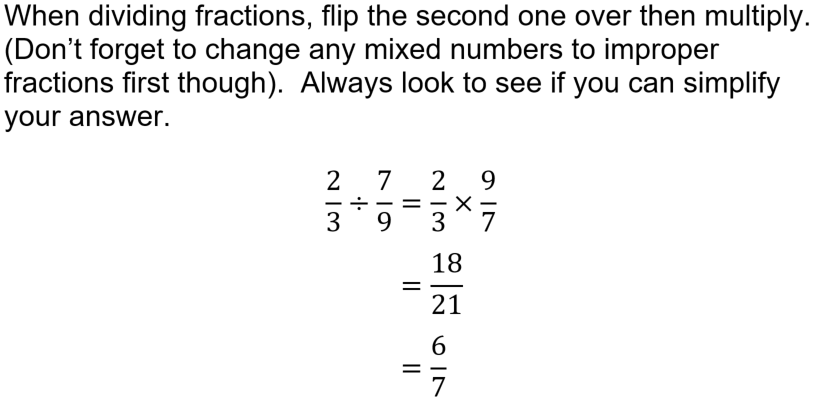9.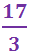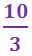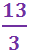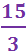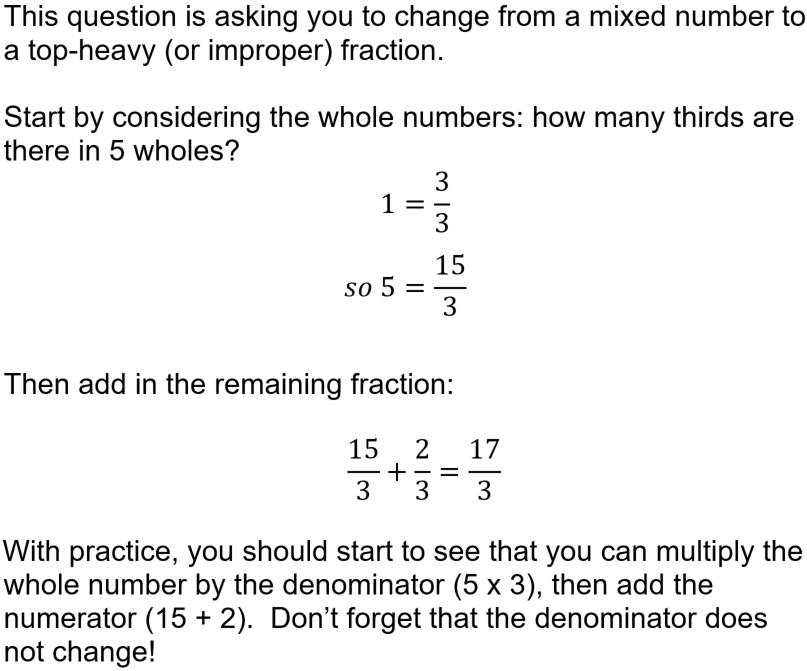10.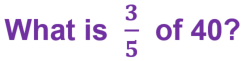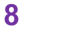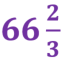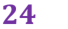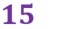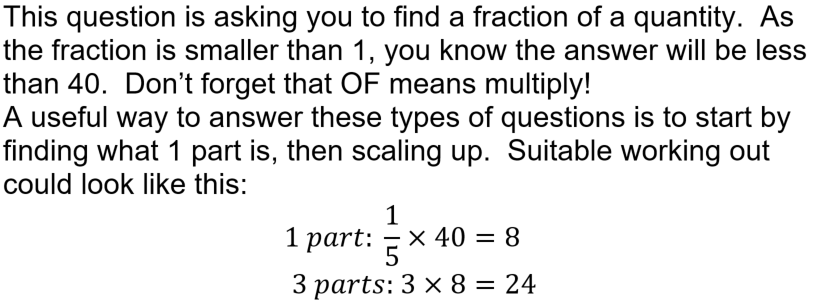Author:  Sally Thompson